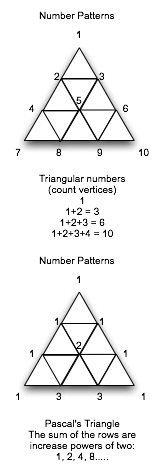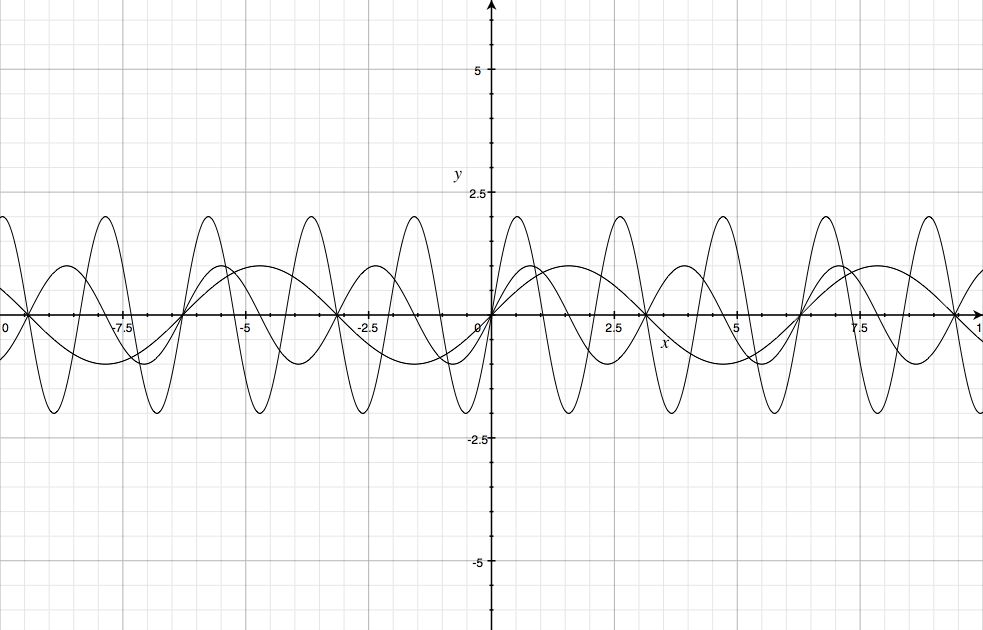Natural Science - Year I

#### Course Materials are always under revision! Weblecture content may change anytime prior to two weeks before scheduled chat session for content.Science Web Assignment for Unit 7

 This Unit's Homework Page History Lecture Science Lecture Lab Parents' Notes

# Science Lecture for Unit 7:Periodicity, Sound, and Wave Properties

##### For Class
• Topic area: Mathematics: Periodicity; Sound: Music
• Terms and concepts to know: Periodic motion, waves, wavelength, proportion
• See historical period(s): Greece

## Proportions and PatternsPythagoras and his followers were responsible for directing attention to the relationships between numbers themselves, quite apart from the ability of numbers to describe or help us keep track of real things. We can imagine, for example, a perfect cone, with different heights. As long as we keep the relative proportions of the cone the same, we can predict the shape, surface of the base, surface area of the whole cone, and its volume.

If we now look at sound moving out from a point source, we see it spread out, the way the base area of the cone spreads out as the distance from the cone point increases. If we have a constant stream of sound from the point of the cone, the sound will have to cover a larger and larger area the further it gets from the point. The intensity of sound reaching any point will go down as base area increases, and the base area increases as the square of the distance from the point. We call this an "inverse square" relationship: intensity is proportional to 1/distance2. Later in this course, we'll discover that many phenomena, including gravity and light, follow the same rule of "spreading out", and can be expressed by an inverse square law.

### Test yourself

Your friend mycroft is standing halfway between you and the loudspeaker playing the soundtrack of your latest favorite movie. Will the music mycroft hears be louder or softer than the music you hear? How much louder of softer?Pythagoras also detected patterns in numbers. The "triangular numbers" mentioned in the History Web Lecture biographies are one of the patterns that he detected. Pythagoras started with 1, and added the next number (2), to get three. If he placed the numbers at the vertices of a triangle, he could continue to generate a triangle by adding the next number in the sequence to the current sum. One of the properties that he found is that the sum of any two adjacent triangular numbers is always a perfect square. For example, we have 1 in the first row, and 3 in the second: 1 + 3 = 4, or 22. We have 3 in the second row and 6 in the third row: 3 + 6 = 9, or 32. Almost 2000 years after Pythagoras, the French mathematician Blaise Pascal showed a similar kind of relationship between numbers set out in a triangular pattern, where the sum of two adjacent numbers produces the next number on the row below.

While these kinds of games can be fun for the mathematically inclined, they have some serious applications to science. Often we look at isolated numerical information without seeing a pattern. If we discover, however, that some quantity is 1 after the first time period, 3 after the second, 6 after the third, we might be inclined to think that the increase follows a mathematical rate related to the triangular number progression, and predict that after 4 hours, we will find 10 of whatever it is we are counting.

Pythagoras' exploration of the value of π and the √2 led him to the conclusion that not all numerical relationships resolve to perfect whole-number ratios. Π and √2 are irrational numbers. We cannot write an exact value for them in any base system (other than their own). You sometimes hear of a computer calculating π to a million places: that's what irrational means. In nature, we find that not only are circles related to their diameter by irrational numbers, and diagonals of squares related to their sides by irrational values, but many rates or changes over time depend on certain types of irrational number relationships. When we try to investigate these and write down data, our accuracy is limited by the fact that we simply cannot write precise numbers for these values. Even if we write symbols, when we calculate, the values we use can only be approximations to the actual number.

### Periodicity

Periodicity is the idea that some event recurs at a more or less precise interval. We've seen how the Egyptians and Babylonians recognized the periodicity of the sun's daily motion, the moon's monthly phases, and even the eclipse patterns of the Saros cycle as examples of some event that was repeated over and over again at a predictable point in time. Pythagoras and those who inherited and expanded his mathematical teachings, Apollonius and Euclid, were aware of mathematical functions that repeat the same values over and over.

One class of these periodic functions forms the basic trigonometric relationships. If we plot the values of one of the functions, such as a sine wave, we discover that it doesn't increase indefinitely or decrease indefinitely as parabolas do. The function y = sine x simply repeats the same values for y over and over in a wave-like pattern as x goes from -infinity to +infinity. The wavelength for each wave is measured from the top of one crest to the top of the next crest, and each wave has three "nodes" or points where the wave pattern crosses the mid point.We can vary the length of time required for a given pattern by changing the value of x. We can vary the height of the wave by multiplying it. But once the wave pattern is set, unless we change these parameters, the pattern will simply repeat indefinitely. This can be very useful in predicting the behavior of "periodic" phenomena!

### Sound

As Pythagoras recognized, it is possible to make a string vibrate with a particular pitch. We can then change the pitch of the string either by shortening or lengthening it, or by changing the input energy.

In the diagram below, the darkest of the three waves makes 1.5 wavelengths within the "box". The lighter "short" wavelength makes 3 wavelengths within the "box". The amplified (high wave crests) wave makes 4.5 wavelengths within the "box". When the wavelengths fit, as they do here, we can get standing waves that produce a constant tone, and we can use those tones to make music. [We'll come back to cover sound in more detail later.]### Harmony and Music

Harmony is the name we give to combinations of pleasing sounds. We generally use musical "scales" or relationships between pitches to make music. Western European music is based on a pitch sequence that has its roots in Pythagoras' mathematics. If we take one of our strings above and cut the distance between frets in half, the frequency of oscillations doubles, giving us a tone one octave higher than the long string. This means that if the note A above middle C is set to 440 Hz, the A an octave higher will have a vibration at 880Hz.

The realization that musical notes have a mathematically proportional relationship led Pythagoras to speculate on other ways in which natural phenomena might match mathematical sequences. He wrote about "the music of spheres", and insisted that all objects in nature fit into whole number sequences of some kind. His ideas greatly influenced the philosopher Plato in the fourth century bce, and during late Antiquity and the Middle Ages, music was considered one of the quadrivium, the four basic mathematical areas of knowledge (the other three were arithmetic, geometry, and astronomy). In the seventeenth century ce, the astronomer Johannes Kepler tried to use Pythagorean proportions to determine the distance of the planets from the sun in his book Harmonium Mundi, or The harmony of the World.

### Study/Discussion Questions

• Why is the idea of proportion important for science?
• What is a numerical series? Why is it useful?
• What is a periodic phenomenon? Give at least two examples that are not astronomical in nature!

### Further Study On your Own (Optional)

• This Conic Section Explorer allow you to change the intersection parameters for the plane and see what happens to the line generated as the intersection. [Requires Adobe Flash]
• Play with this animation, which lets you adjust the tension and dampling in the string to see how waves are generated.